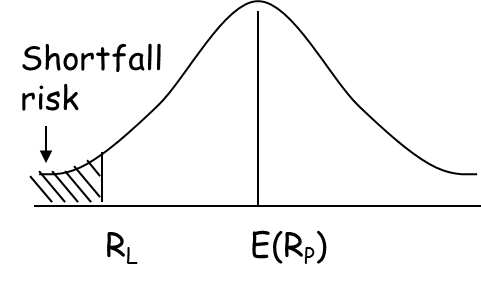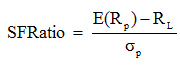# Short-fall Risk, Safety-first Ratio, and Optimal Portfolio Selection

Shortfall Risk

Shortfall risk refers to the probability that a portfolio will not exceed the minimum return level (target return, benchmark return). Shortfall-risk is more consistent with the investors’ intuitive perception of risk in that it focusses more on the real economic risk of an investor. On the other hand, the standard deviation is rather a measure of the volatility of financial assets. Shortfall risk is also known as downside risk.

The following diagram illustrates shortfall risk. As we can see, it is the risk that the portfolio value will fall below some minimum acceptable level, RL.Safety-first ratio

Roy’s Safety first criterion states that the optimal portfolio minimizes the probability that portfolio return, RP, falls below RL.

According to this criteria, we select the portfolio with the highest safety first ratio (SFRatio).Example

An investor has a minimum threshold of 2%. He has to make a choice between two portfolios A and B.

 Portfolio A Portfolio B E(R) 10% 15% s 20% 25%

According to Roy’s Safety-first criteria, which portfolio should he choose?

SFRatio of Portfolio A = (10-2)/20 = 0.40

SFRatio of Portfolio B = (15-2)/25 = 0.52

According to safety-first criteria, we should select portfolio B. Minimum threshold is 0.40 standard deviations away for portfolio A while it is 0.52 standard deviations away for portfolio B.

# R Programming Bundle: 25% OFF

Get our R Programming - Data Science for Finance Bundle for just $29$39.
Get it now for just \$29